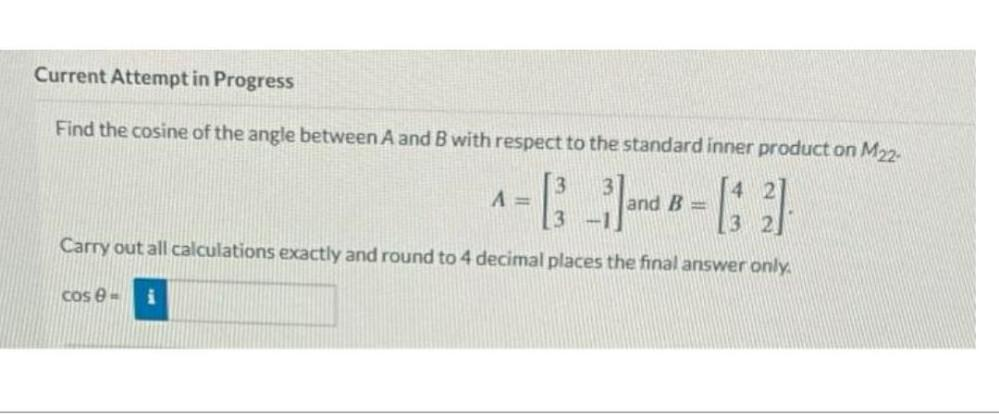Question:

# Find the cosine of the angle between A and B with respect to the standard inner product on M22 A =[3 3] and B = [4 2] [3 -1] [3 2] Carry out all calculations exactly and roundFind the cosine of the angle between A and B with respect to the standard inner product on M22 A =[3 3] and B = [4 2] [3 -1] [3 2] Carry out all calculations exactly and round to 4 decimal places the final answer only